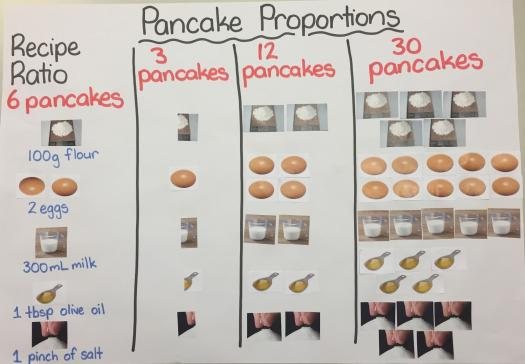# What Do You Know About Ratio And Proportion

10 Questions | Total Attempts: 117SettingsEvery day we use proportions. Whether it's in the kitchen, when we decide to paint our home or when we measure the medicine we're about to take. Without even knowing it, we are able to respect dosages, and follow recipes to the letter. Now, do you think you can pass our quiz? Complete this small quiz and see how well you do.

• 1.
What's a proportion?
• A.

It's an equation with a ratio on each side.

• B.

It's a ratio.

• C.

It's the quantity of something.

• D.

It's the measurement of something.

• 2.
If there are 9 red apples and 3 green apples, the ratio of the red apples to the green apples is 9/3=3. How can you interpret this data?
• A.

It means that there are 2 times more red apples than green apples.

• B.

It means that there are 6 times more red apples than green apples.

• C.

It means that there are 3 times more red apples than green apples.

• D.

It means that there are 9 times more red apples than green apples.

• 3.
If there are 3 boys and 6 girls and the ratio of boys to girls is 3/6. What does it mean?
• A.

That there are three times as many boys as girls.

• B.

That there are twice as many boys as girls.

• C.

That there are half as many girls as boys.

• D.

That there are half as many boys as girls.

• 4.
What's a ratio?
• A.

It's a comparison of 2 numbers using division.

• B.

It's a comparison of 2 numbers using addition.

• C.

It's a comparison of 3 numbers using division.

• D.

It's a comparison of 2 numbers using subtraction.

• 5.
How do you write the ratio of a to b?
• A.

A/b with b different than 0.

• B.

A + b

• C.

A * b with b different than 0.

• D.

A - b with a different than 0.

• 6.
How is the ratio of 3 or more quantities called?
• A.

A discontinued ratio

• B.

A complex ratio

• C.

A triple ratio

• D.

A continued ratio

• 7.
What's the ratio of 4 to 8 to 12?
• A.

Continued ratio 4+8+12

• B.

Triple ratio 4:8:12

• C.

Discontinued ratio 4:8:12

• D.

Continued ratio 4:8:12

• 8.
A classroom has 50 students and the ratio of females to males is to 2 to 3. How many students are males?
• A.

30 males.

• B.

20 males

• C.

60 males

• D.

40 males

• 9.
Complete the following : In general, when two fractions are-------------, they form a proportion.
• A.

Greater than 2

• B.

Different

• C.

Equivalent

• D.

Greater than 5

• 10.
A girl who is 3 feet tall can cast a shadow on the ground that is 7 feet long. How tall is a girl who can cast a shadow that is 14 feet long?
• A.

6 feet tall.

• B.

8 feet tall.

• C.

10 feet tall.

• D.

12 feet tall.

Related TopicsBack to top